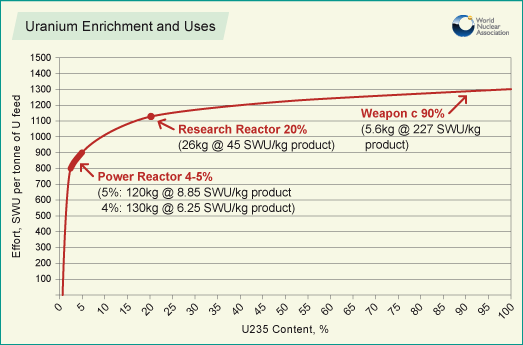# Separative work unitFigure 1. Graph showing increase in SWU with increase in 235U concentration. Power reactors here depicts Light Water reactors (e.g. PWR and BWR's).

The Separative Work Unit (SWU) is a unit that defines the effort required in the uranium enrichment process, in which uranium-235 and -238 are separated. The separative work unit is measured in units of kg (kilograms) and can then be manipulated to determine cost per SWU and kWh (kilowatt hour) per SWU (it is not work in the traditional physics sense).

Natural uranium is 0.711% 235U and needs to be enriched to between 3 and 5% for use in a light water reactor (a BWR or a PWR). In order to do this, the uranium undergoes an enrichment process that requires an input of separative work. This is due to the second law of thermodynamics which states that the entropy (disorder) of any isolated system must increase; but because separated isotopes represent more order (lower entropy) than mixed isotopes, effort must be put in to enrich the uranium.

## Calculating SWU

The amount of uranium required to be fed into the enrichment plant in order to obtain a desired amount of enriched product depends on the desired enrichment of the product, the original enrichment of the feed and the enrichment of the depleted uranium or the 'tails'. Knowing this, a mass balance equation using these three masses and their respective percent concentrations can be written:

............. (Equation 1)

Where xF is the concentration of the feed uranium and MF is the mass of the feed uranium, xP is the concentration of the product uranium and MP is the mass of the product uranium, xT is the concentration of the tails and MT is the mass of the tails.

Applying conservation of mass (MF = MP + MT) and manipulating the equation a little bit we're left with:

............. (Equation 2)

The concentration of the feed uranium will almost always be 0.711%, which is the concentration of natural uranium, therefore as long as the desired concentration of product and tails along with desired mass of product are known, mass of feed can be determined.

Separative work can be expressed in terms of a function V(x) known as the value function, given by:

............. (Equation 3)

Where x is the enrichment concentration.

The SWU can then be determined using the masses of each uranium concentration found using equation 2 and the value function found for each respective concentration:

............. (Equation 4)

For a better understanding of SWU, a online SWU calculator can be used.

## Example Calculation

You want to make 20 kg of uranium enriched to 3.8% 235U by weight. Your tailing fraction is 0.2% by weight. You can produce 1 kg SWU for 50 kWhr at a cost of $130.75 per 1 kg SWU. How much feed uranium do you need? Here we use Equation 2: Therefore we would need 140.9 kg of feed uranium at a concentration of 0.711% in order to produce 20 kg of 3.8% enriched uranium. How much energy does it take to make this uranium and what is the cost? Here we first use Equation 3 for each of the concentration percentages and plug what we get into Equation 4: Do this for the other two concentrations and find the values equal: V(xF)= 4.869, V(xT)= 6.188. Then plug these three value functions into Equation 4 to find: We can then multiply this number by both the kWh and cost per SWU to find our two answers: And Therefore it would take 6090.355 kWh to produce this uranium at a cost of$15926.27.

## Authors and Editors

Bethel Afework, Haydon Armstrong, Jordan Hanania, Celeste Pomerantz, Jason Donev
Last updated: May 18, 2018
Get Citation